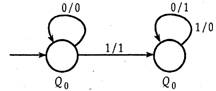# GATE | GATE-CS-2005 | Question 63

• Last Updated : 28 Jun, 2021

The following diagram represents a finite state machine which takes as input a binary number from the least significant bit.Which one of the following is TRUE?
(A) It computes 1’s complement of the input number
(B) It computes 2’s complement of the input number
(C) It increments the input number
(D) It decrements the input number

Explanation: The given finite state machine takes a binary number from LSB as input.

The given FSM remains unchanged till first ‘1’ . After that it takes 1’s complement of rest of the input string.

We assume the input string to be ‘110010’ .
Thus, according to the FSM, output is ‘001110’ .

2’s complement of ‘110010’ = 1’s complement of ‘110010’ + 1 = 001101 + 1 = 001110
Thus, the FSM computes 2’s complement of the input string.

Hence, option (B) is correct.

Please comment below if you find anything wrong in the above post.

Quiz of this Question

Attention reader! Don’t stop learning now.  Practice GATE exam well before the actual exam with the subject-wise and overall quizzes available in GATE Test Series Course.

Learn all GATE CS concepts with Free Live Classes on our youtube channel.

My Personal Notes arrow_drop_up